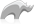##### [ regexp ]

Type

No description available.

• Syntax
• Methods
• Traits
``No syntax description available at this time.``
###### Has methods:
• `regexp->oncompare(s::string)`
• `regexp->oncreate(p0::string, p1::string, p2::string, p3::boolean)`
• `regexp->input()`
• `regexp->replacepattern()`
• `regexp->findpattern()`
• `regexp->ignorecase()`
• `regexp->input=(p0::string)`
• `regexp->replacepattern=(p0::string)`
• `regexp->findpattern=(p0::string)`
• `regexp->ignorecase=(p0::string)`
• `regexp->setinput(p0::string)`
• `regexp->setreplacepattern(p0::string)`
• `regexp->setfindpattern(p0::string)`
• `regexp->setignorecase(p0::string)`
• `regexp->output()`
• `regexp->appendreplacement(p0::string)`
• `regexp->matches()`
• `regexp->matches(p0::integer)`
• `regexp->private_replaceall()`
• `regexp->appendtail()`
• `regexp->groupcount()`
• `regexp->matchposition(p0::integer)`
• `regexp->matchposition()`
• `regexp->matchesstart()`
• `regexp->matchesstart(p0::integer)`
• `regexp->private_replacefirst()`
• `regexp->private_split()`
• `regexp->find()`
• `regexp->find(p0::integer)`
• `regexp->matchstring(p0::integer)`
• `regexp->reset(-input = ?, -find = ?, -replace = ?, -ignorecase = ?)`
• `regexp->replaceall(replace::string)`
• `regexp->replaceall(-input = ?, -find = ?, -replace = ?, -ignorecase = ?)`
• `regexp->replacefirst(-input = ?, -find = ?, -replace = ?, -ignorecase = ?)`
• `regexp->split(-input = ?, -find = ?, -replace = ?, -ignorecase = ?)`
• `regexp->findall(-input = ?, -find = ?, -ignorecase = ?)`
• `regexp->findcount(-input = ?, -find = ?, -ignorecase = ?)`
• `regexp->findfirst(-input = ?, -find = ?, -ignorecase = ?)`
###### Has traits:
• `any`
• `trait_asString`
• Beginner
• Intermediate

### Remove anything but digits from a string containing a phone number.

It's a good habit to use back-ticks instead of quotes to enclose find and replace patterns in a [regexp] object since it won't need special handling of escape characters.

Code

``regexp(-find = `\D+`, -input = 'Phone: 905 251-6169', -ignorecase) -> replaceall``

Result

`-> 9052516169`

Task at hand, find the name that's in-between the `<h1></h1>` tags of a given html snippet. There can be both line feeds and tabs that we don't want as part of the result.

To extract the name value we do a two step operation. First replace any kind of line feeds or tabs with nothing. Next step is to weed out the content between the `<h1></h1>` tag.

The [regexp] methods we use is [replaceall], [input], [findpattern], [find] and [matchstring]. To remove possible extra space before and after the resulting string we trim it.

Code

``````var(myhtml = '<!DOCTYPE html>
<html lang="en">
<title>Testing regexp</title>
<body>
<h1>
LassoSoft Inc - The Rhino from Canada
</h1>
</body>
</html>')

var(reg_exp = regexp(-find = `(\r\n)+|\r+|\n+|\t+`, -replace = ``, -input = \$myhtml))

var(strippedhtml = \$reg_exp -> replaceall)

\$reg_exp -> input = \$strippedhtml

\$reg_exp -> findpattern = `<h1>(.*?)</h1>`
var(final = (\$reg_exp -> find ? \$reg_exp -> matchstring(1) | 'Not found') -> trim &)

\$final
``````

Result

`-> LassoSoft Inc - The Rhino from Canada`Please note that periodically LassoSoft will go through the notes and may incorporate information from them into the documentation. Any submission here gives LassoSoft a non-exclusive license and will be made available in various formats to the Lasso community.